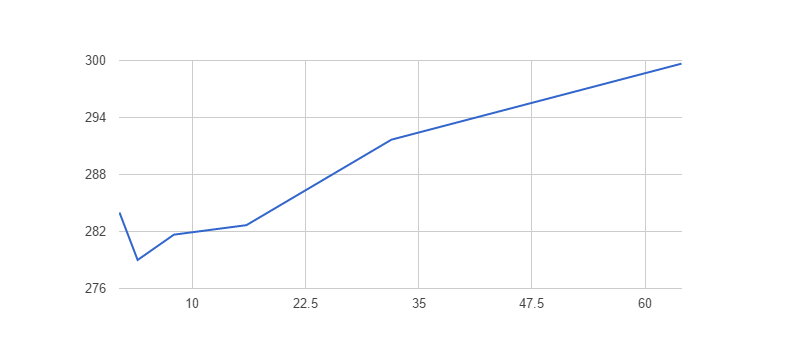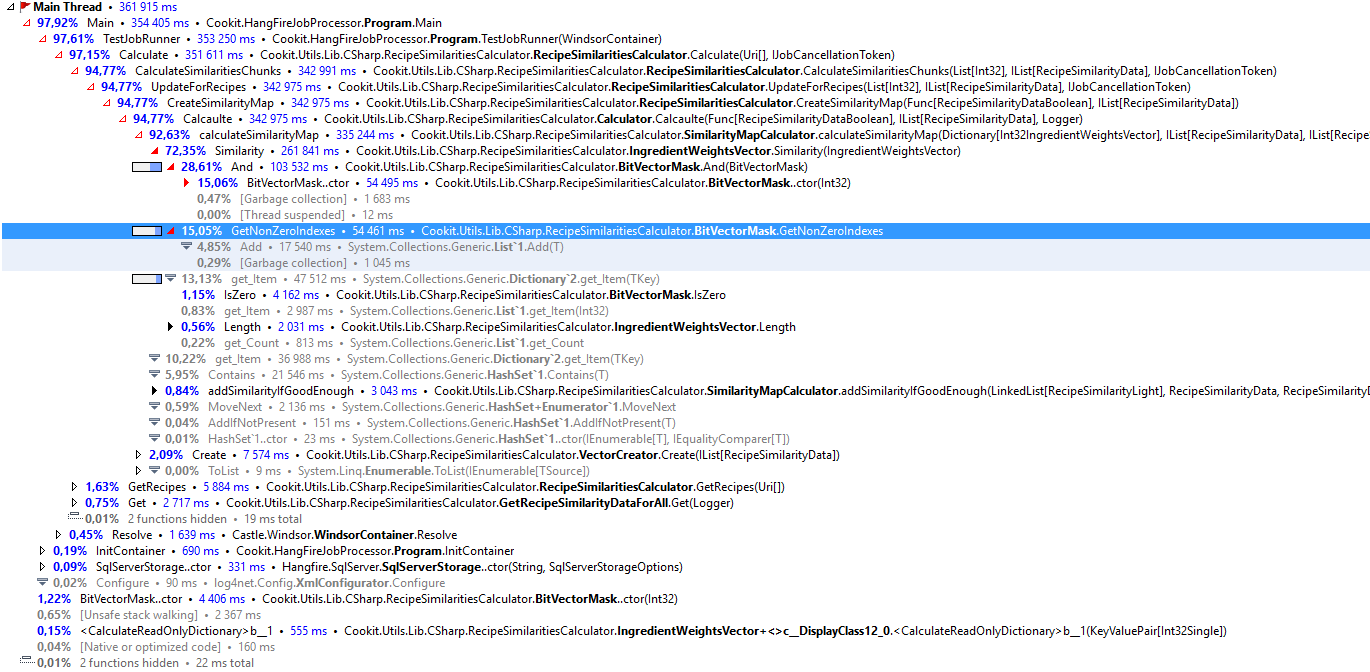# Dividing a bit in two for performance

## December 20, 2016

This post is an analysis of a very interesting optimization proposed by Nicholas Frechette in the comments under the previous post and t0rakka on Reddit. They proposed to use one of the oldest tricks in performance cookbook - divide and conquer. Well, it did not turn out as I expected.

## Recap

The last implementation of `GetNonZeroIndexes` looked like this:

``````        public IList<int> GetNonZeroIndexes()
{
List<int> ret= new List<int>();
for (var i = 0; i < _array.Length; i++)
{
var a = _array[i];
if (a != 0UL)
{
if ((a & 1UL) != 0UL) ret.Add(i * 64 + 0);
if ((a & 2UL) != 0UL) ret.Add(i * 64 + 1);
if ((a & 4UL) != 0UL) ret.Add(i * 64 + 2);
if ((a & 8UL) != 0UL) ret.Add(i * 64 + 3);
.
.
.
.
.
if ((a & 1152921504606846976UL) != 0UL) ret.Add(i * 64 + 60);
if ((a & 2305843009213693952UL) != 0UL) ret.Add(i * 64 + 61);
if ((a & 4611686018427387904UL) != 0UL) ret.Add(i * 64 + 62);
if ((a & 9223372036854775808UL) != 0UL) ret.Add(i * 64 + 63);
}
}
return ret;
}
``````

How can this get better?

## Divide and conquer

### Theory

The above approach is a brute force approach. Why? I am iterating over the long and checking for every bit if it is set. This is wasteful since most of them are not set (remember those are sparse arrays). The better approach would be to test multiple bits at once. How? Bit masks - as always :)

Using bit masks and the `AND` operation I could check the first 32 bits (from 64 that `ulong` has):

``````0000000011111111 <- masc
0001100110001001 <- value

0000000010001001 <- after AND
``````

If any bit in this range is set then the result will be greater than zero. Simple as that.

### Implementation

I could replace `GetNonZeroIndexes` with this code:

``````public IList<int> GetNonZeroIndexes()
{
var ret = new List<int>();
for (var i = 0; i < _array.Length; i++)
{
var a = _array[i];
if (a != 0UL) //is any bit set?
{
if ((a & BitVectorMaskConsts._0_32) > 0) //is any of the 32 least significant bits set?
{
if ((a & BitVectorMaskConsts._0_16) > 0) //is any of the 16 least significant bits set?
{
if ((a & BitVectorMaskConsts._0_8) > 0) //is any of the 8 least significant bits set?
{
if ((a & BitVectorMaskConsts._0_4) > 0) //is any of the 4 least significant bits set?
{
if ((a & 1UL) != 0UL) ret.Add(i*64 + 0);
if ((a & 2UL) != 0UL) ret.Add(i*64 + 1);
if ((a & 4UL) != 0UL) ret.Add(i*64 + 2);
if ((a & 8UL) != 0UL) ret.Add(i*64 + 3);
}
if ((a & BitVectorMaskConsts._4_8) > 0){
.
.
.
.

}
}
}
}
}
return ret;
}
``````

### Complexity

What divide and conquer is doing is simulating a binary tree consisting of 64 nodes.

Since my tree is fully balanced (equal number of nodes on the left and right) its max depth is equal to `log n`. With `n` equal to a number of leafs in the tree. I am doing a brute force iteration over the last 4 elements so my number of leafs is 64/4 = 16. This means that I’ll be doing from 5 to 9 checks in the worst case. Looks promising.

## Performance results

How did it affect performance? The last best sample run took 297 seconds. With divide and conquer it takes 283 seconds. This translates to:

``````(283 / 2199) * 182184 ~ 6,5 hours (starting from 34 hours, and 6,8 in the best run)
``````

So I am doing even an order of magnitude fewer operations than before and all I get is 15 seconds? Maybe if I go to leaf size of 2 it will be better? I will be doing less so it should be faster? Even more, let’s plot how execution time is dependent on leaf size

### Leaf size vs. time

Below is a table comparing leaf size to execution time (they are an average of 3 runs).

Size of the chunk Execution time St.dev
64 299 seconds 2.3
32 277 seconds 3.5
16 296 seconds 3.5
8 293 seconds 2.3
4 283 seconds 6.5
2 297 seconds 4.3## Making sense of it all

Why did my execution time not go down as expected? Couple reasons:

## Things changed

I’ve started from 968 seconds for the test run, and most of this time was spend in `Similarity`. Now if I look at the profiler:`Similarity` is still using the most CPU, but it is not the `GetNonZeroIndexes` that is the hogger. I’m just slowly getting closer and closer to the minimum execution time.

## Why leaf size of 2 is slower than leaf size of 4?

Execution in current processors in not linear. They execute instructions in advance hoping that they will guess the flow. When they are right it is very fast, when it is a miss performance suffers greatly. It is called branch prediction.

This situation leads to the fact that sorting and then processing the array may be faster then just processing, or calculating the minimum of two numbers is faster using bit operations then using if.

My guess is that two and four leaf implementation suffers mostly from that.

Hi, I'm Szymon Warda. I write code, design IT systems, write this blog, tweet and speak at conferences. If You want to know more go here, or follow me:

### MongoDB vs RavenDB

A comparison between MongoDB and RavenDB Continue reading

#### Tips, tricks, and good practices for Data-Driven Testing. Part 2.

Published on September 09, 2019

#### Tips, tricks, and good practices for Data-Driven Testing. Part 1.

Published on September 03, 2019